# Best approximations, sequence of

A sequence,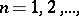of numbers, where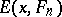is the best approximation of an elementof a normed linear spaceby elements of an-dimensional subspace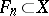, with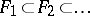, so that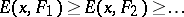. Usually,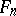is the linear span of the firstelements of some fixed system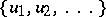of linearly independent elements of.

In the case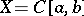and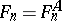is the subspace of algebraic polynomials of degree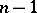, sequences of best approximations were first considered in the 1850s by P.L. Chebyshev; the fact that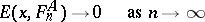for any function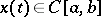was established in 1885 by K. Weierstrass. In the general case, the relation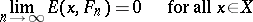is always satisfied when the union of the subspaces,is everywhere dense in,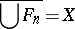(essentially, this is an equivalent statement). However, the sequencemay converge to zero arbitrarily slowly. This follows from a theorem of Bernstein: Ifis a sequence of subspaces of dimensionof a normed linear space, such thatand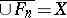, then, for any monotone decreasing null sequenceof non-negative real numbers, there exists ansuch that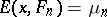,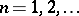. In the function spacesand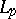, the rate at which a sequence of best approximations tends to zero depends both on the system of subspacesand on the smoothness characteristics of the approximated function(the modulus of continuity, the existence of derivatives up to a specific order, etc.), and it can be estimated in terms of these characteristics. Conversely, knowing the rate of convergence to zero of the sequence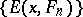, one can draw conclusions with respect to the smoothness of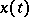(see Approximation of functions, direct and inverse theorems).